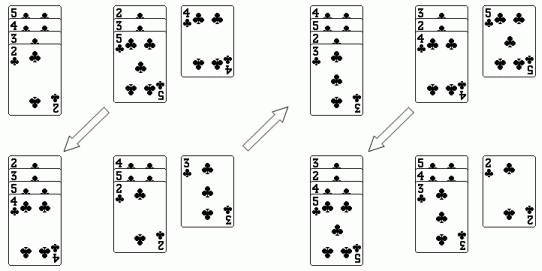# How to make a permutation of a card problem and compute its order?

Risto arranged card deck cards first by suits and when by numerical values. After that he started to deal cards to piles such that every pile contained equal number of cards. As he had no cards, he collected the piles and started to deal the deck in the similar process. It took time but Risto continued doealing. Suddenly the cards were on their original order.

Program knows the number of cards, number of piles, and the number of cards to be put on every pile. The task is to compute how many times Risto must collect the cards such that the cards are on their original order.

Cards have been dealt one at time and the cards have been put one the piles on the same order. As one has dealt every card, piles are gathered together such that the first pile goes to the most bottom in the pile. After that the cards in the new order will be piled and combined ia a similar way. Finally the order of cards will be as in the start.

In the next picture one can see a process if there are four cards and two piles. In the first pine one always adds two cards and on the second pile one adds one card. In this case a pile contains three cards and on the second deal there remains only one card that goes to the first pile.After four arrangements the order of the cards is as in the beginning. This means it takes four rounds to get cards to their original order.

How can I solve the problem by computer if the data of cards are as in https://www.ohjelmointiputka.net/tied... ? The first number is the number of cards, then the number of piles and the next the number of cards one adds to every pile.

Every line contains - number of cards - number of rounds to get cards into the original order.

For example, the answer to the example is 4 4

I think one could make some kind of permutation and compute its order. But how can I implement it?

edit retag close merge delete

Sort by » oldest newest most voted

I hope i understood the rule of the game. (If something went wrong on the road, please mention the bad permutation, and the expected one, i will correct the code.)

Here is the sage implementation of the order of the one step permutation implemented by dealing once the set of cards. The lines

Every line contains - number of cards - number of rounds to get cards into the original order.

are of course a joke. Let us concentrate on the main task, computing the order.

def getOrder(N, pile_len_list, show_one_step_permutation=False):
"""Compute
"""
n_piles = len(pile_len_list)

cards = [1..N]
piles = [ [] for _ in range(n_piles) ]    # so far, and we prepend
k_card = -1
k_pile = -1

while cards:    # to be dealt
k_pile += 1
k_pile = k_pile % n_piles

pile_cards_number = min( len(cards), pile_len_list[k_pile] )
# print( f"........ cards = {cards}" )
pile_cards = cards[:pile_cards_number]
cards = cards[pile_cards_number:]
piles[k_pile] = pile_cards[::-1] + piles[k_pile]
# print( f"... cards = {cards} k_pile = {k_pile} piles = {piles} "
#        f"pile_cards_number = {pile_cards_number} pile_cards = {pile_cards}\n" )

permuted_list = []
for k in list(range(n_piles))[::-1]:
permuted_list += piles[k]
if show_one_step_permutation:
print( f"ONE STEP PERMUTATION = {permuted_list}" )
return lcm( [ len(cycle)
for cycle in Permutation(permuted_list).to_cycles() ] )


Now, using the sage interpreter with the above defined function, we can ask for...

sage: getOrder(4, [2,1], True)
ONE STEP PERMUTATION = [3, 4, 2, 1]
4
sage:


corresponding to the case in the OP. There, instead of $2\clubsuit$, $3\clubsuit$, $4\clubsuit$, $5\clubsuit$, we use a simpler notation and the "new cards" 1, 2, 3, 4. After dealing one step, we get the order $4\clubsuit$, $5\clubsuit$, $3\clubsuit$, $2\clubsuit$, corresponding the "new cards" new order 3, 4, 2, 1. So position 1, goes to position 4, which goes to position 2, which goes to position 3, which goes to position 1.

sage: p = Permutation( [3, 4, 2, 1] ).inverse()
sage: p(1)
4
sage: p(4)
2
sage: p(3)
1
sage: p(2)
3
sage: p(3)
1


I had to insert the inverse method, convention... but the order does not change. We take the cycles, then compute their lengths, then take their lcm.

sage: getOrder( 9, [1, 2, 3], True )
....:
ONE STEP PERMUTATION = [6, 5, 4, 9, 8, 3, 2, 7, 1]
20
sage: p = Permutation( [6, 5, 4, 9, 8, 3, 2, 7, 1] )
sage: p.inverse().to_cycles()
[(1, 9, 4, 3, 6), (2, 7, 8, 5)]


which corresponds to one of the lines in the link in the OP. The one step permutation is a product of two disjoined cycles of lengths $5$ and $4$, so the needed order is $20$.

In more dramatic cases, we get:

sage: getOrder( 77777, [1, 2, 3, 5, 8, 13, 21, 34, 55,] )
359539078526124596396388
sage: _.factor()
2^2 * 3^2 * 7^2 * 13 * 23 * 29 * 41 * 47 * 59 * 73 * 83 * 3593 * 9497

more